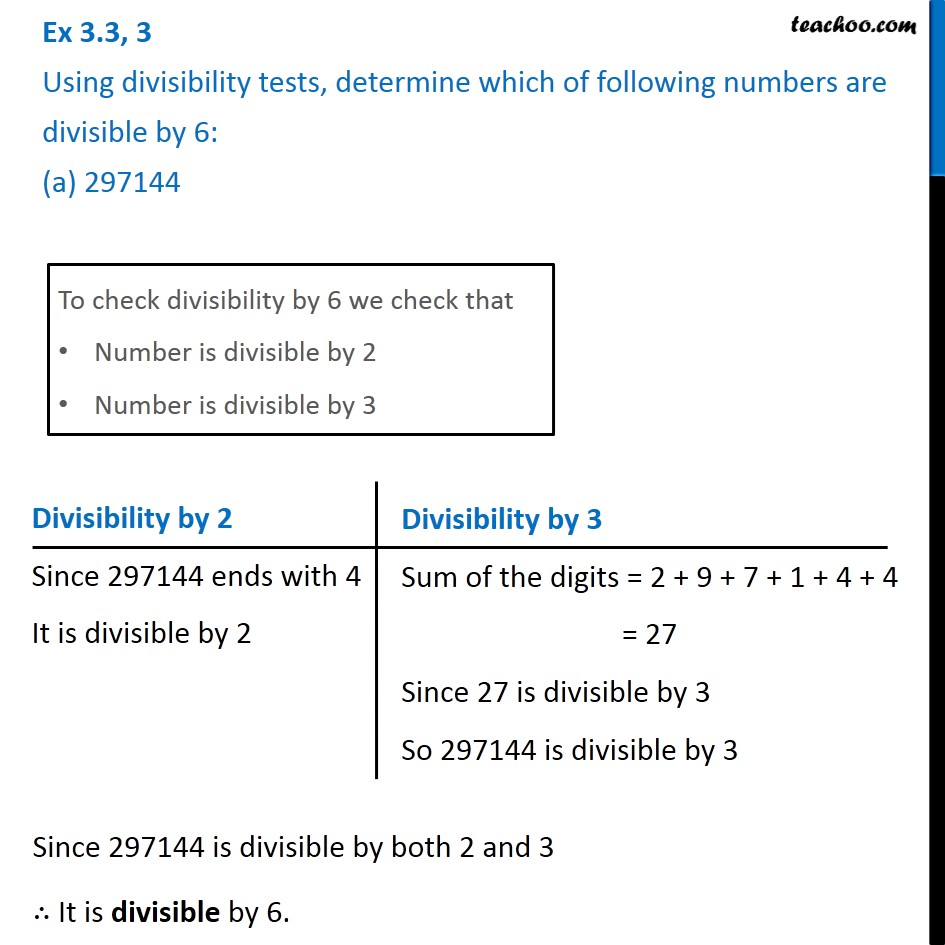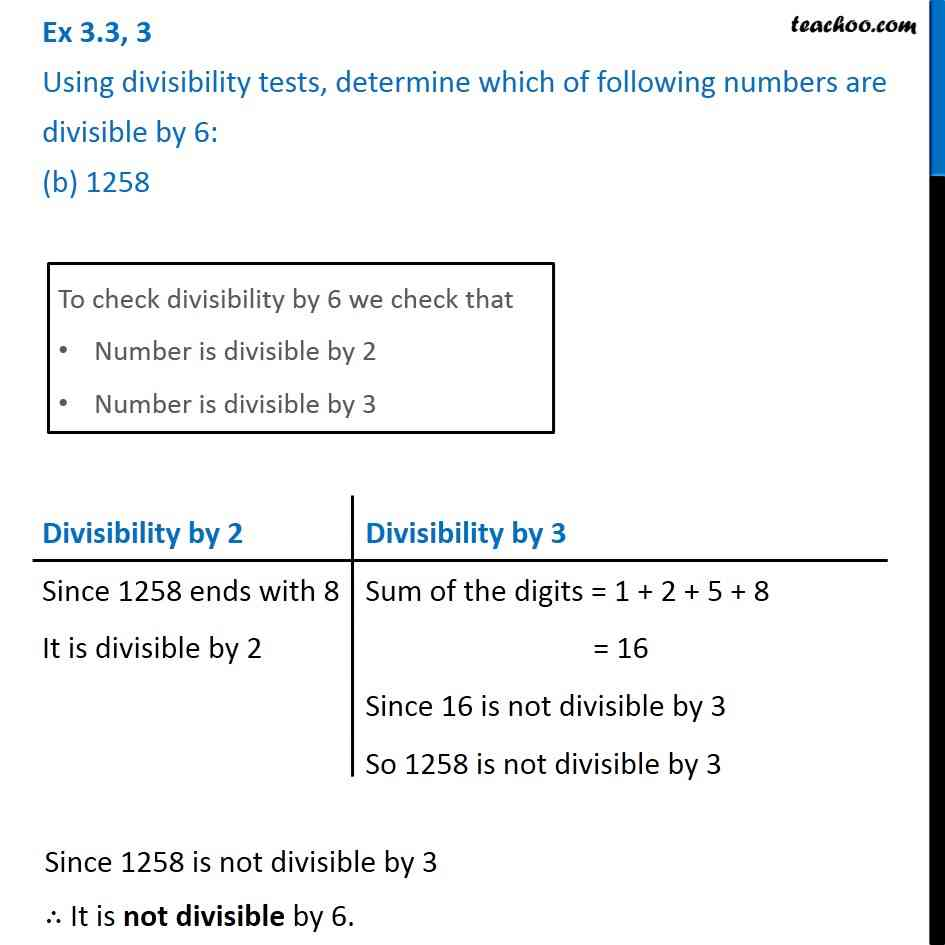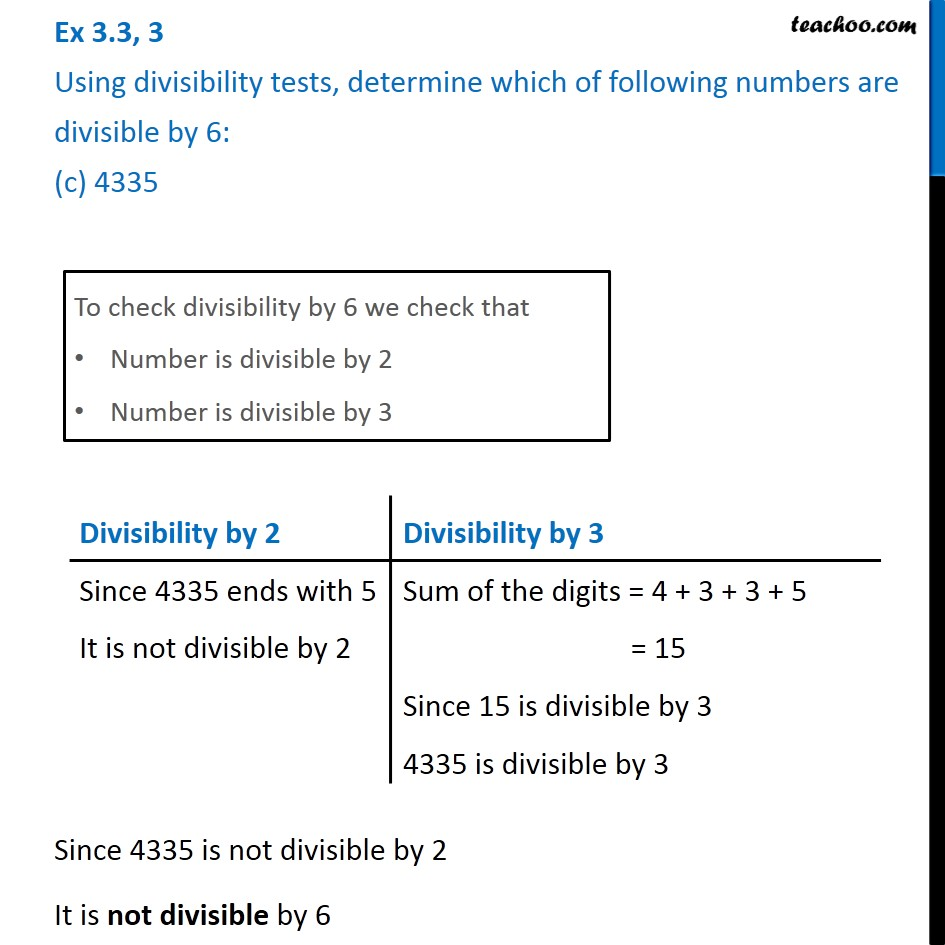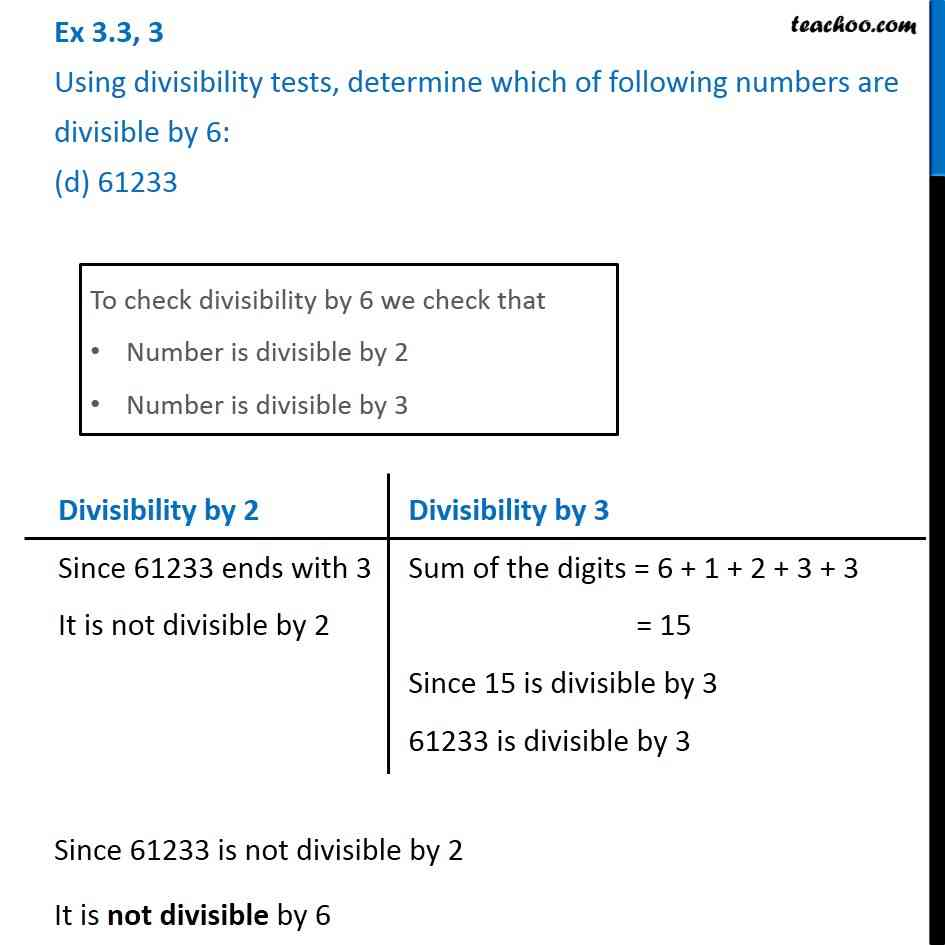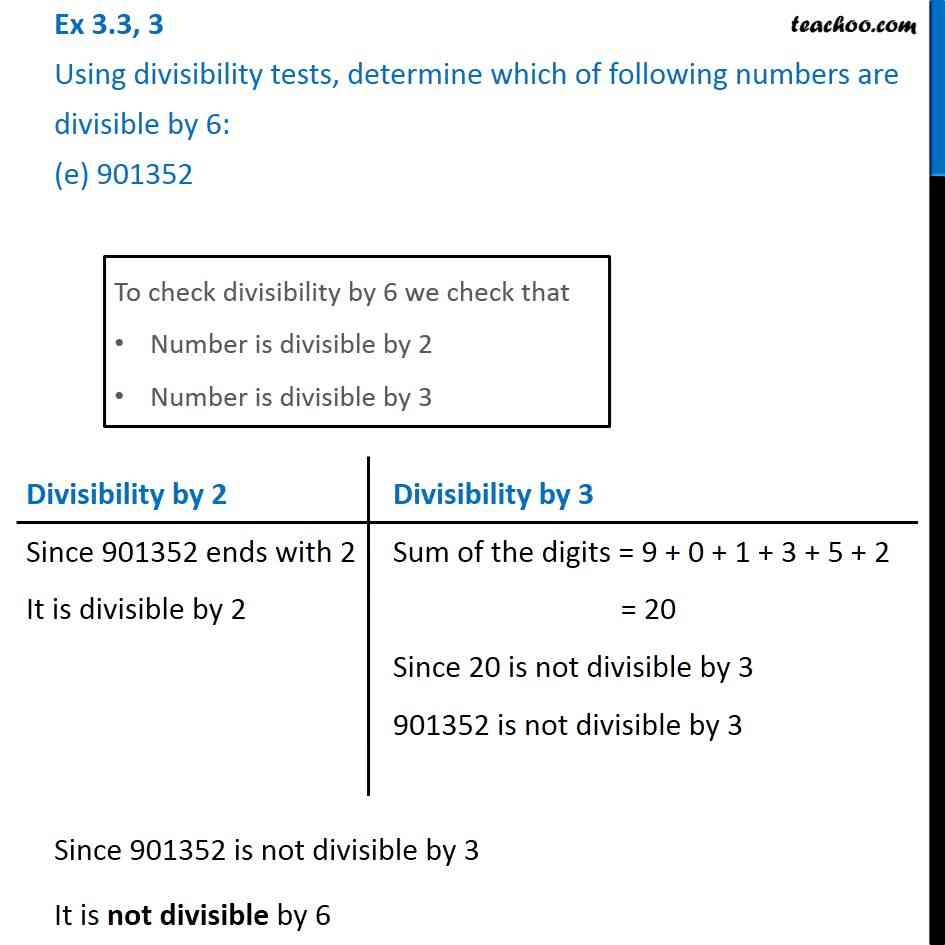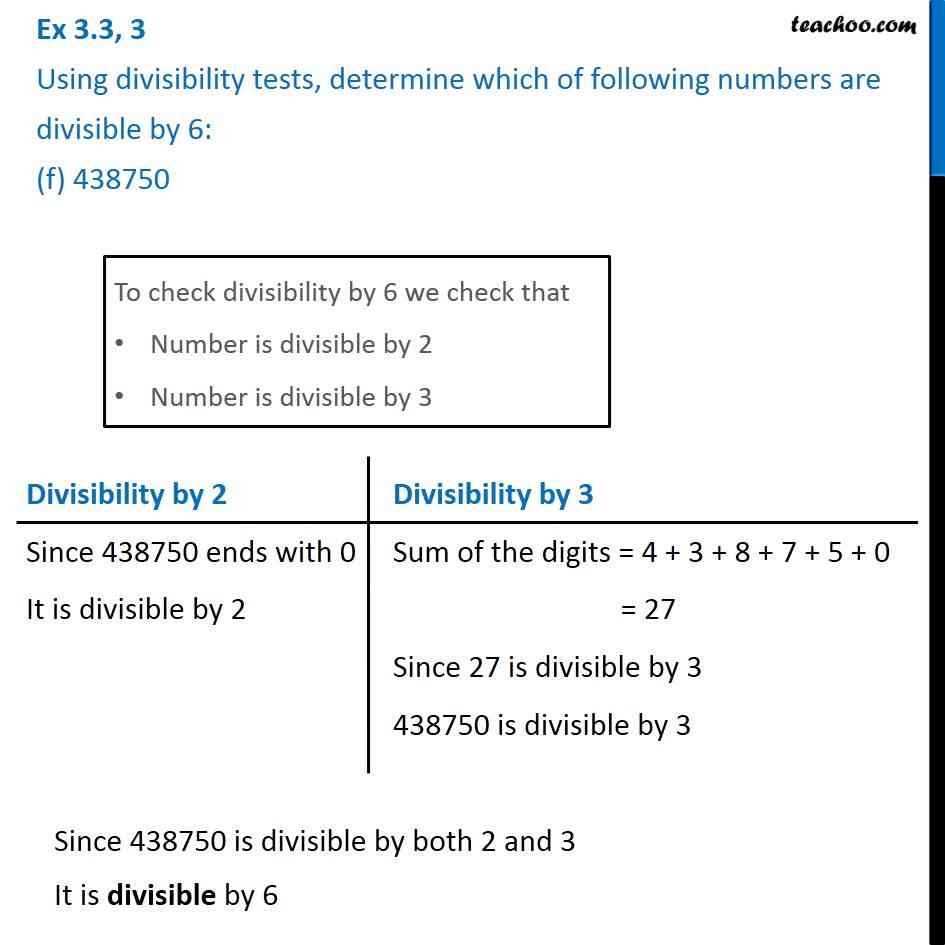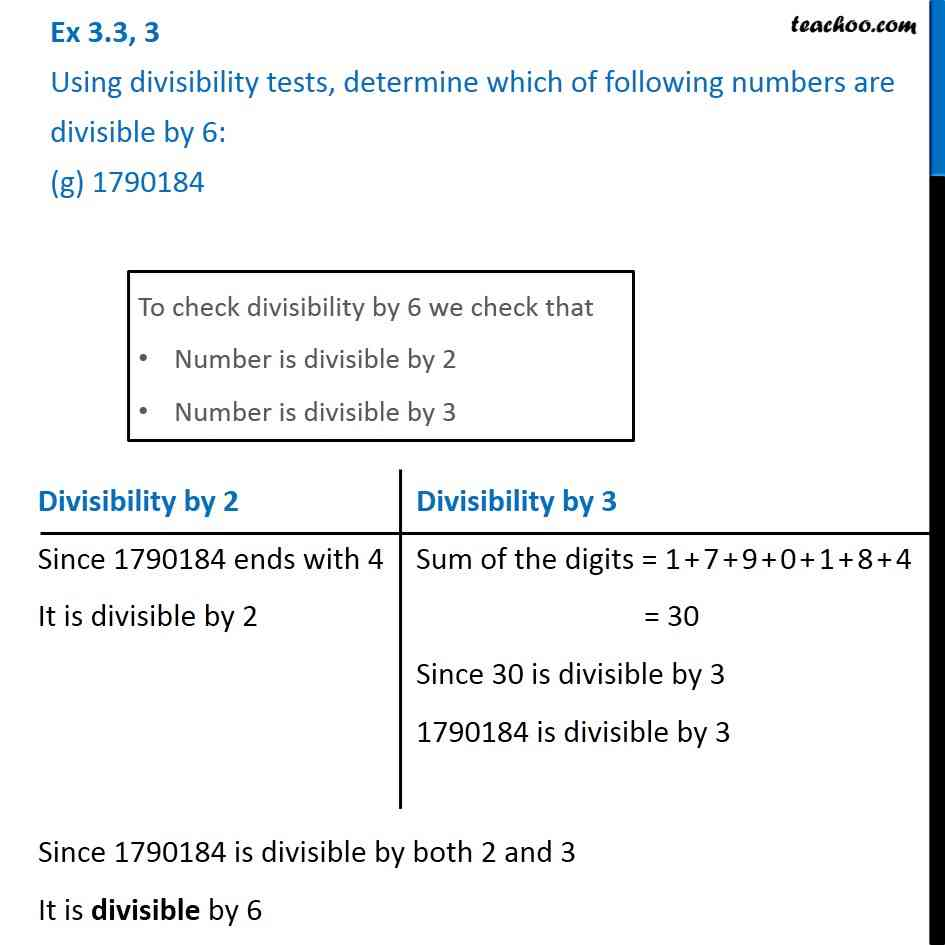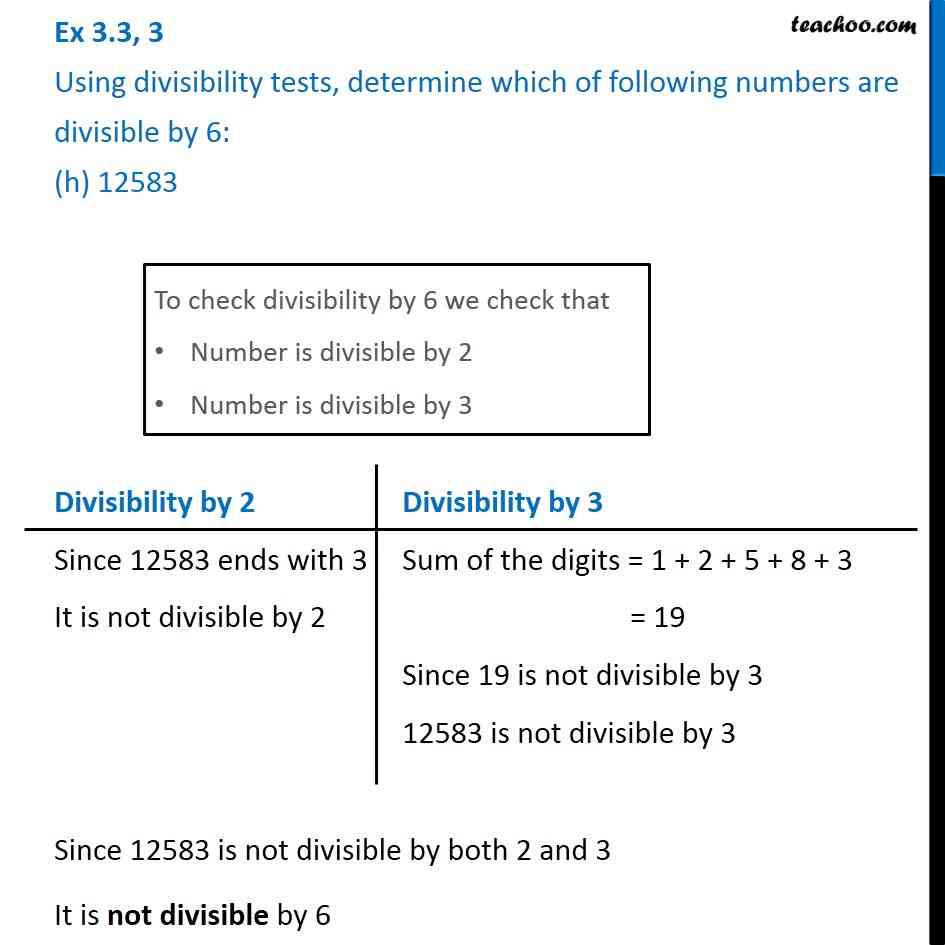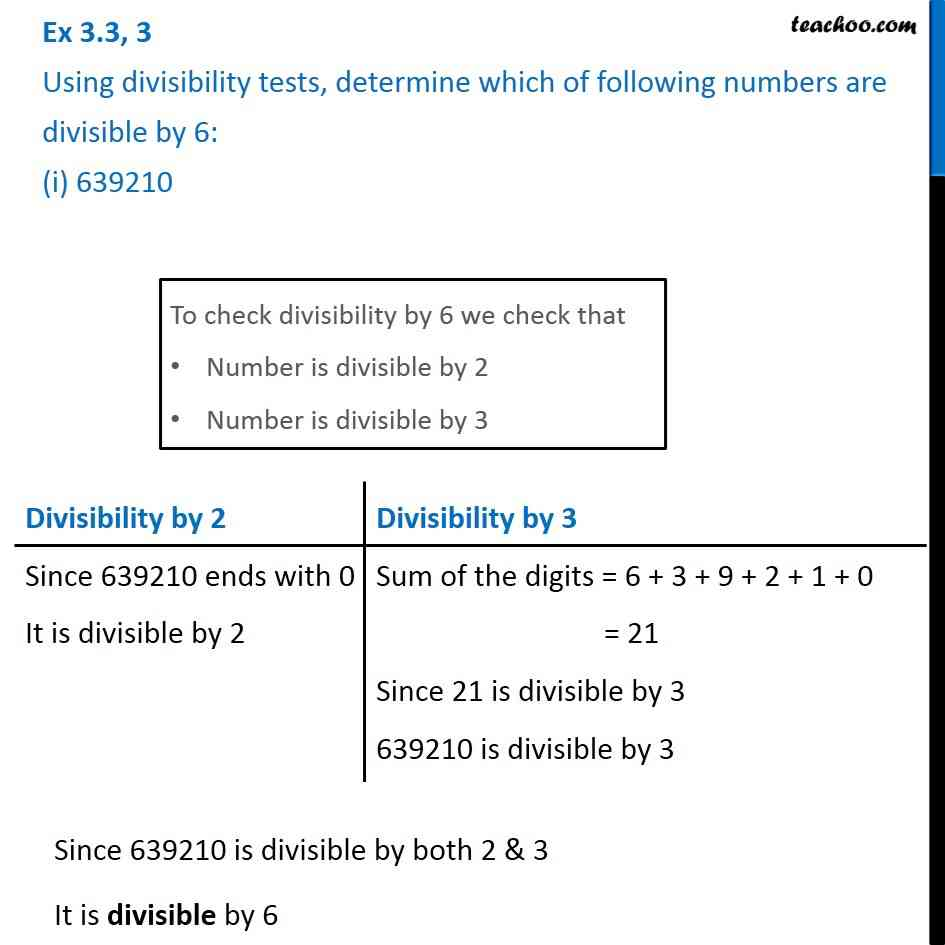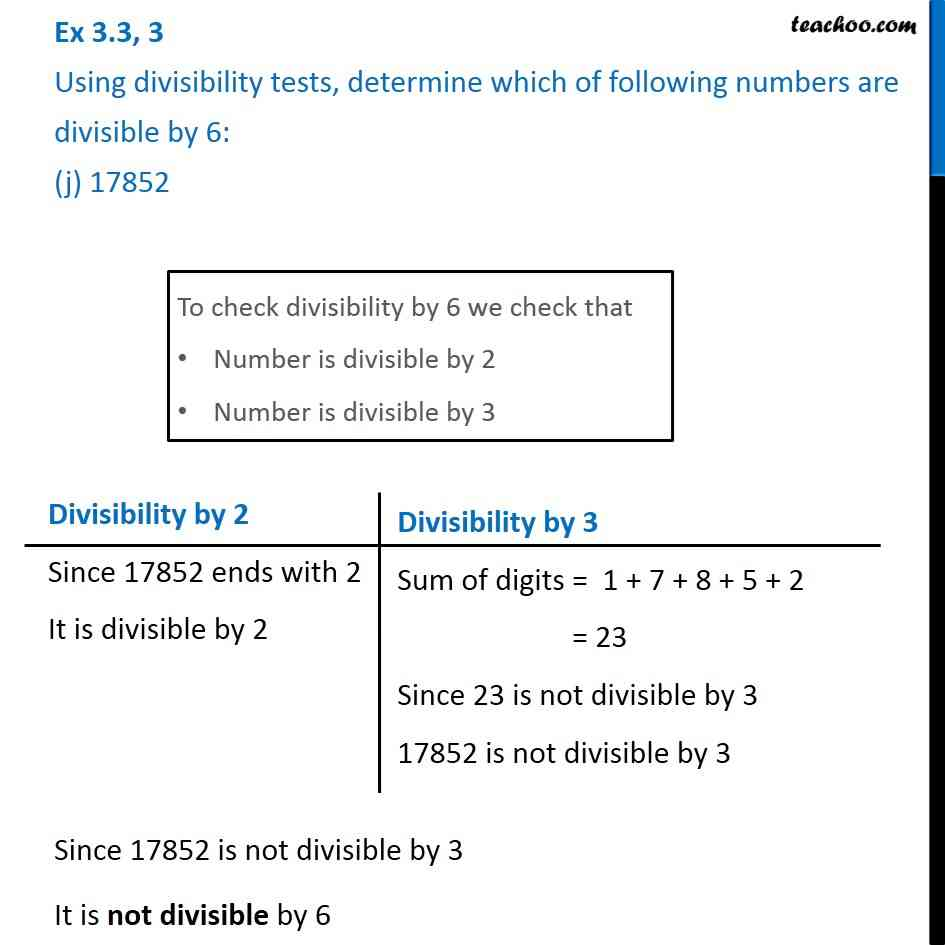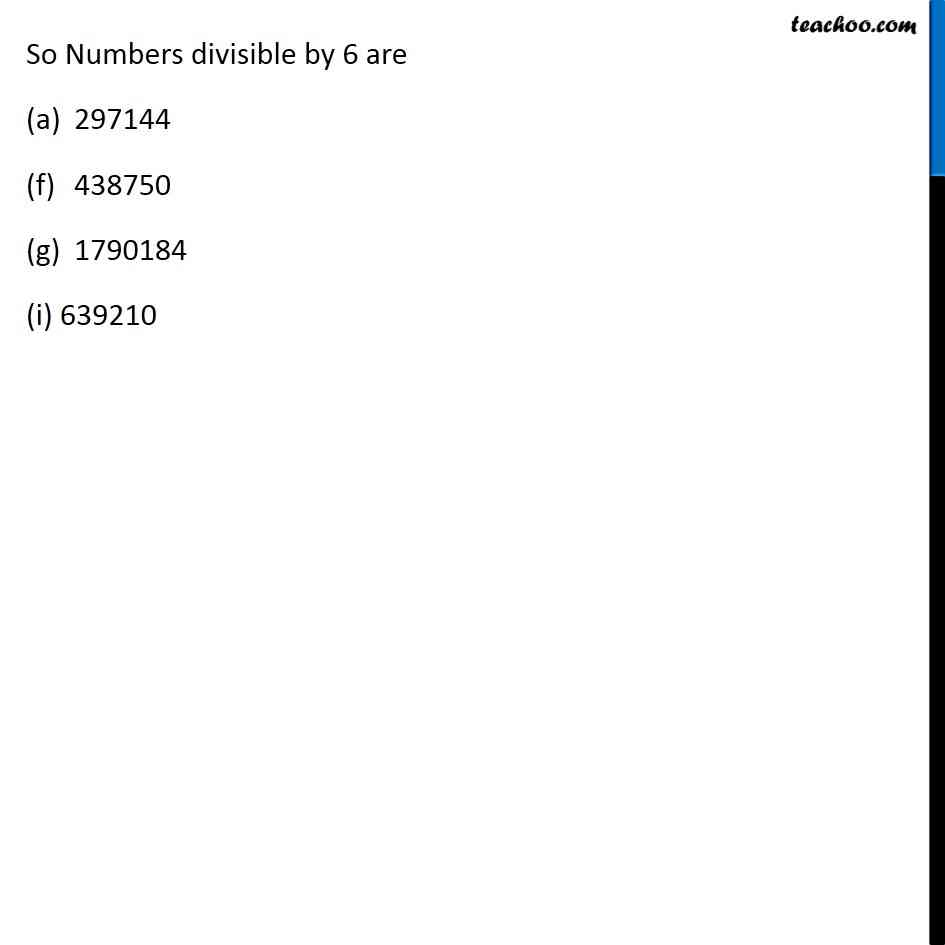Subscribe to our Youtube Channel - https://you.tube/teachoo

1. Chapter 3 Class 6 Playing with Numbers
2. Serial order wise
3. Ex 3.3

Transcript

Ex 3.3, 3 Using divisibility tests, determine which of following numbers are divisible by 6: (a) 297144 To check divisibility by 6 we check that Number is divisible by 2 Number is divisible by 3 Divisibility by 2 Since 297144 ends with 4 It is divisible by 2 Divisibility by 3 Sum of the digits = 2 + 9 + 7 + 1 + 4 + 4 = 27 Since 27 is divisible by 3 So 297144 is divisible by 3 Since 297144 is divisible by both 2 and 3 ∴ It is divisible by 6. Ex 3.3, 3 Using divisibility tests, determine which of following numbers are divisible by 6: (b) 1258 To check divisibility by 6 we check that Number is divisible by 2 Number is divisible by 3 Divisibility by 2 Since 1258 ends with 8 It is divisible by 2 Divisibility by 3 Sum of the digits = 1 + 2 + 5 + 8 = 16 Since 16 is not divisible by 3 So 1258 is not divisible by 3 Since 1258 is not divisible by 3 ∴ It is not divisible by 6. Ex 3.3, 3 Using divisibility tests, determine which of following numbers are divisible by 6: (c) 4335 To check divisibility by 6 we check that Number is divisible by 2 Number is divisible by 3 Divisibility by 2 Since 4335 ends with 5 It is not divisible by 2 Divisibility by 3 Sum of the digits = 4 + 3 + 3 + 5 = 15 Since 15 is divisible by 3 4335 is divisible by 3 Since 4335 is not divisible by 2 It is not divisible by 6 Ex 3.3, 3 Using divisibility tests, determine which of following numbers are divisible by 6: (d) 61233To check divisibility by 6 we check that Number is divisible by 2 Number is divisible by 3 Since 61233 is not divisible by 2 It is not divisible by 6 Ex 3.3, 3 Using divisibility tests, determine which of following numbers are divisible by 6: (e) 901352 To check divisibility by 6 we check that Number is divisible by 2 Number is divisible by 3 Divisibility by 2 Since 901352 ends with 2 It is divisible by 2 Divisibility by 3 Sum of the digits = 9 + 0 + 1 + 3 + 5 + 2 = 20 Since 20 is not divisible by 3 901352 is not divisible by 3 Since 901352 is not divisible by 3 It is not divisible by 6 Ex 3.3, 3 Using divisibility tests, determine which of following numbers are divisible by 6: (f) 438750To check divisibility by 6 we check that Number is divisible by 2 Number is divisible by 3 Divisibility by 2 Since 438750 ends with 0 It is divisible by 2 Divisibility by 3 Sum of the digits = 4 + 3 + 8 + 7 + 5 + 0 = 27 Since 27 is divisible by 3 438750 is divisible by 3 Since 438750 is divisible by both 2 and 3 It is divisible by 6 Ex 3.3, 3 Using divisibility tests, determine which of following numbers are divisible by 6: (g) 1790184To check divisibility by 6 we check that Number is divisible by 2 Number is divisible by 3 Divisibility by 2 Since 1790184 ends with 4 It is divisible by 2 Divisibility by 3 Sum of the digits = 1 + 7 + 9 + 0 + 1 + 8 + 4 = 30 Since 30 is divisible by 3 1790184 is divisible by 3 Since 1790184 is divisible by both 2 and 3 It is divisible by 6 Ex 3.3, 3 Using divisibility tests, determine which of following numbers are divisible by 6: (h) 12583To check divisibility by 6 we check that Number is divisible by 2 Number is divisible by 3 Divisibility by 2 Since 12583 ends with 3 It is not divisible by 2 Divisibility by 3 Sum of the digits = 1 + 2 + 5 + 8 + 3 = 19 Since 19 is not divisible by 3 12583 is not divisible by 3 Since 12583 is not divisible by both 2 and 3 It is not divisible by 6 Ex 3.3, 3 Using divisibility tests, determine which of following numbers are divisible by 6: (i) 639210Divisibility by 2 Since 639210 ends with 0 It is divisible by 2 Divisibility by 3 Sum of the digits = 6 + 3 + 9 + 2 + 1 + 0 = 21 Since 21 is divisible by 3 639210 is divisible by 3 Since 639210 is divisible by both 2 & 3 It is divisible by 6 Ex 3.3, 3 Using divisibility tests, determine which of following numbers are divisible by 6: (j) 17852Divisibility by 2 Since 17852 ends with 2 It is divisible by 2 Divisibility by 3 Sum of digits = 1 + 7 + 8 + 5 + 2 = 23 Since 23 is not divisible by 3 17852 is not divisible by 3 Since 17852 is not divisible by 3 It is not divisible by 6 So Numbers divisible by 6 are 297144 438750 1790184 (i) 639210

Ex 3.3